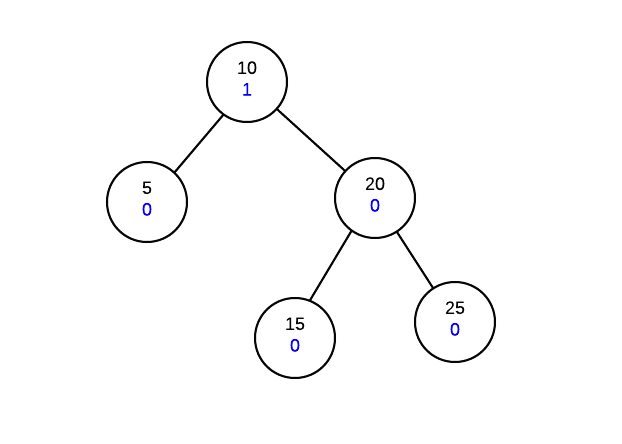# Height Balance

AVL trees work by ensuring that the tree is height balanced after an operation. If we were to have to calculate the height of a tree from any node, we would have to traverse its two subtrees making this impractical. Instead, we store the height balance information of every subtree in its node. Thus, each node must not only maintain its data and children information, but also a height balance value.

The height balance of a node is calculated as follows:

height balance = height of right - height of left
of node      subtree             subtree


The above formula means that if the right subtree is taller, the height balance of the node will be positive. If the left subtree is taller, the balance of the node will be negative.